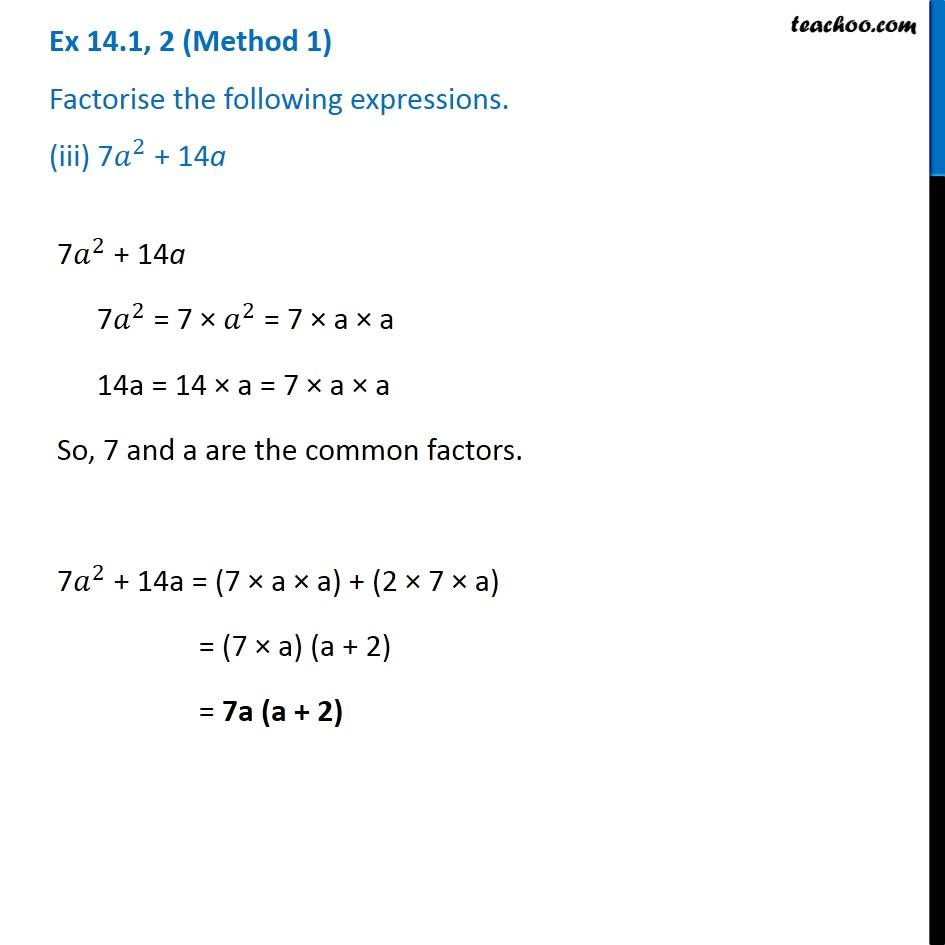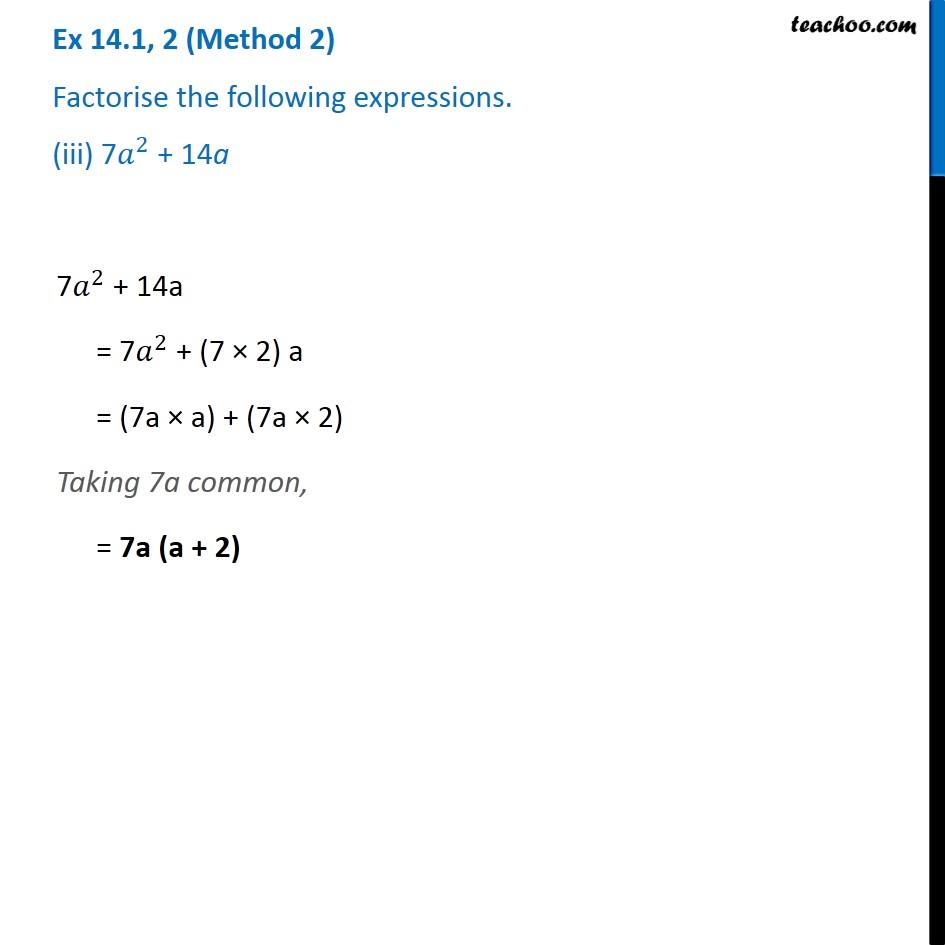Ex 14.1

Chapter 14 Class 8 Factorisation
Serial order wiseGet live Maths 1-on-1 Classs - Class 6 to 12

### Transcript

Ex 14.1, 2 (Method 1) Factorise the following expressions. (iii) 7𝑎^2 + 14a 7𝑎^2 + 14a 7𝑎^2 = 7 × 𝑎^2 = 7 × a × a 14a = 14 × a = 7 × a × a So, 7 and a are the common factors. 7𝑎^2 + 14a = (7 × a × a) + (2 × 7 × a) = (7 × a) (a + 2) = 7a (a + 2) Ex 14.1, 2 (Method 2) Factorise the following expressions. (iii) 7𝑎^2 + 14a 7𝑎^2 + 14a = 7𝑎^2 + (7 × 2) a = (7a × a) + (7a × 2) Taking 7a common, = 7a (a + 2)